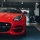Home
IT Knowledge
Inspiration
Languages
EN

# Java 8 - count distinct elements in List

0 points
Created by:Explosssive
339

In this article, we would like to show you how to count distinct elements in List working with Java 8.

Quick solution:

``````List<String> list = Arrays.asList("A", "B", "C", "B", "A");

long count = list.stream().distinct().count();

System.out.println(count);  // 3``````

## Practical example

In this example, we use Stream `distinct()` and `count()` methods to count distinct elements in the `letters` list.

``````import java.util.*;

public class Example {

public static void main(String[] args) {
// create list of strings
List<String> letters = Arrays.asList("A", "B", "C", "B", "A");

// count distinct elements in list
long count = letters.stream().distinct().count();

// print result
System.out.println("Number of distinct elements : " + count);
}
}``````

Output:

``The number of distinct elements : 3``